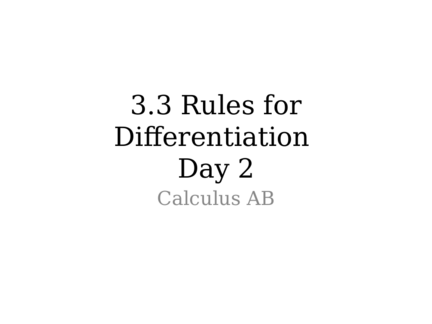# Product Rule and Quotient RuleContributed by:Here, we will discuss the product and quotient rule of differentiation.
1. 3.3 Rules for
Day 2
Calculus AB
2. Derivative Rules
• Product Rule:
• Quotient Rule:
3.
4. Example 1: Find f’(x)
5. Example 2: Differentiate
6. Example 3: Let y = uv. Find y’(2) if
u(2)=3, u’(2)=-4, v(2)=1, and v’(2)=2
7. Example 4: Find the first four
derivatives of y
8. Assignment
Pg. 124
13, 14, 16-21, 23, 24, 28, 31-35, 55,57
Pg. 126
55-58